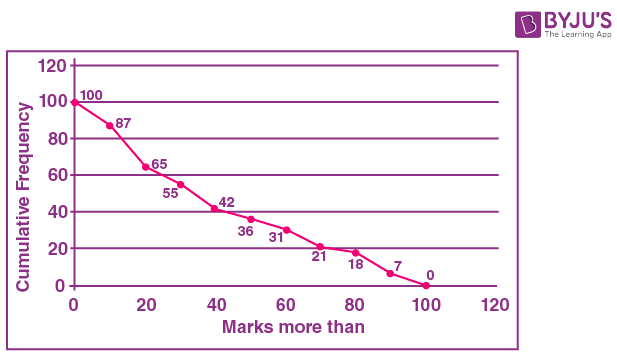# Frank Solutions for Class 10 Maths Chapter 23 Graphical Representation

Frank Solutions for Class 10 Maths Chapter 23 Graphical Representation, are provided here. Our solution module employs numerous shortcut tips and practical examples to explain all the exercise questions in a simple and easily understandable language. Our expert tutors at BYJU’S have simplified the difficult problems into simple steps, which can be easily solved by students at ease. These solutions will help you in obtaining knowledge and strong command over the subject. Download pdf of Frank Solutions for Class 10 Maths Chapter 23 in their respective links.

Chapter 23 – Graphical Representation, a graph is defined as a pictorial representation or a diagram that represents data or values in an organised manner. As the chapter is about Graphs, here we learn how to plot the graph, draw the histogram graph and read the details from the graph and further solve the same.

## Download the PDF of Frank Solutions for Class 10 Maths Chapter 23 Graphical Representation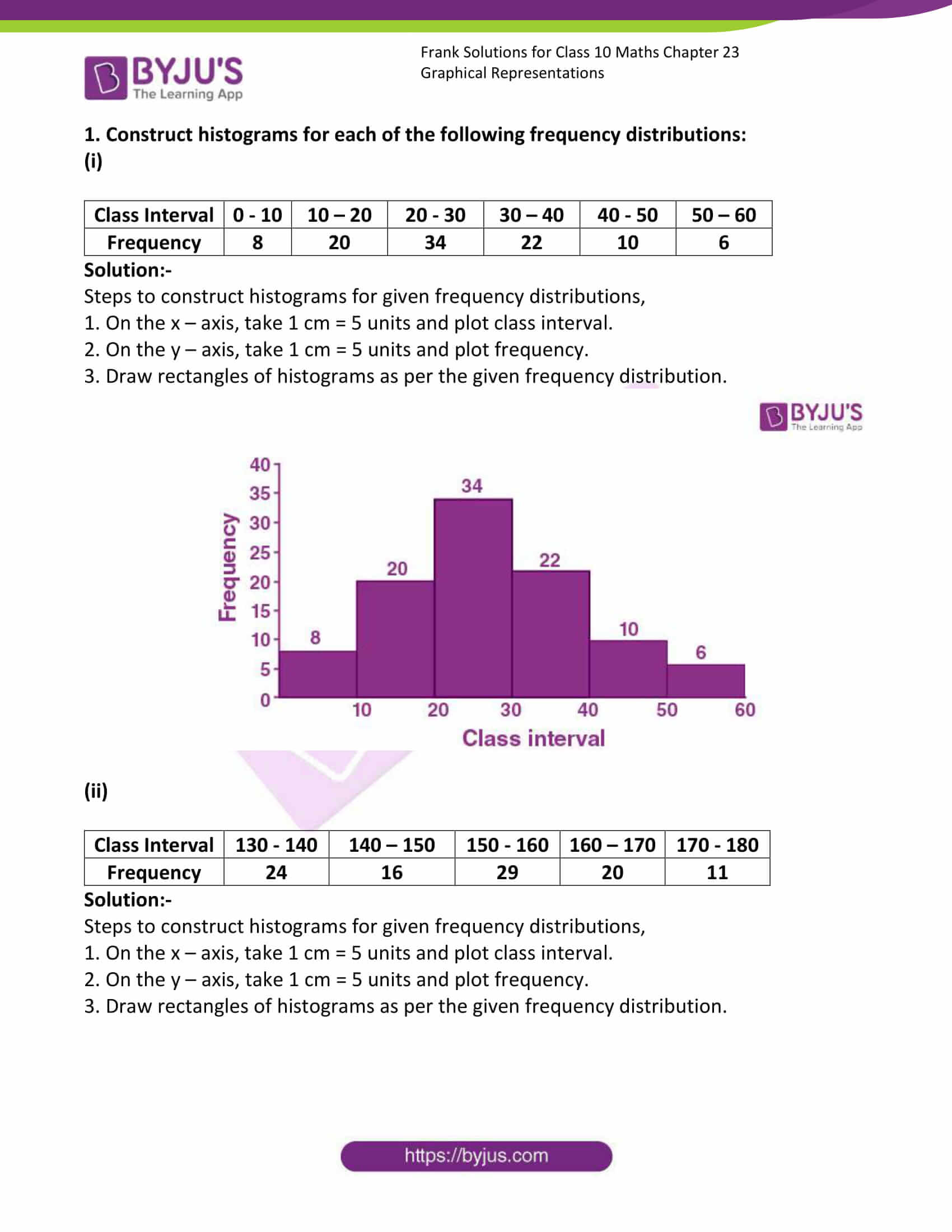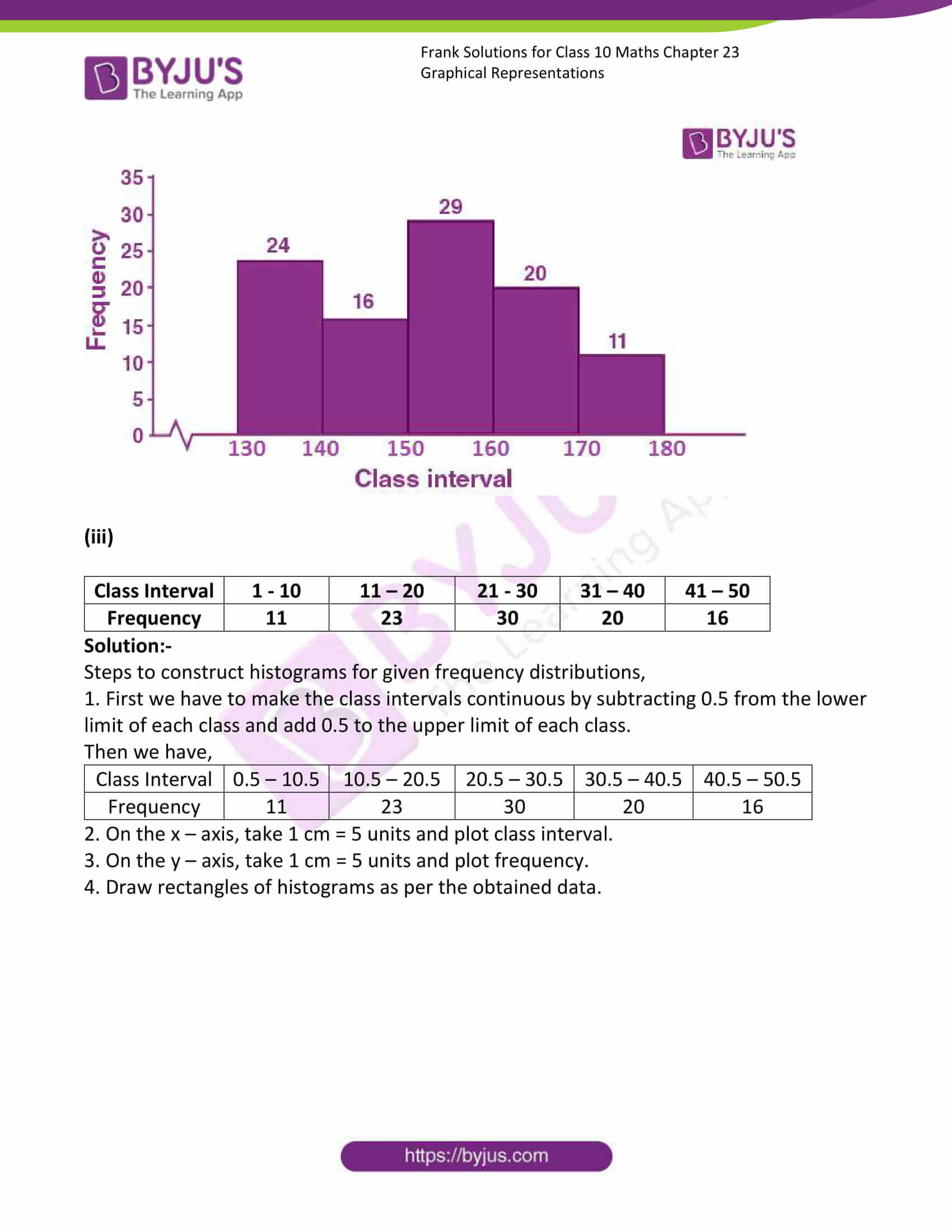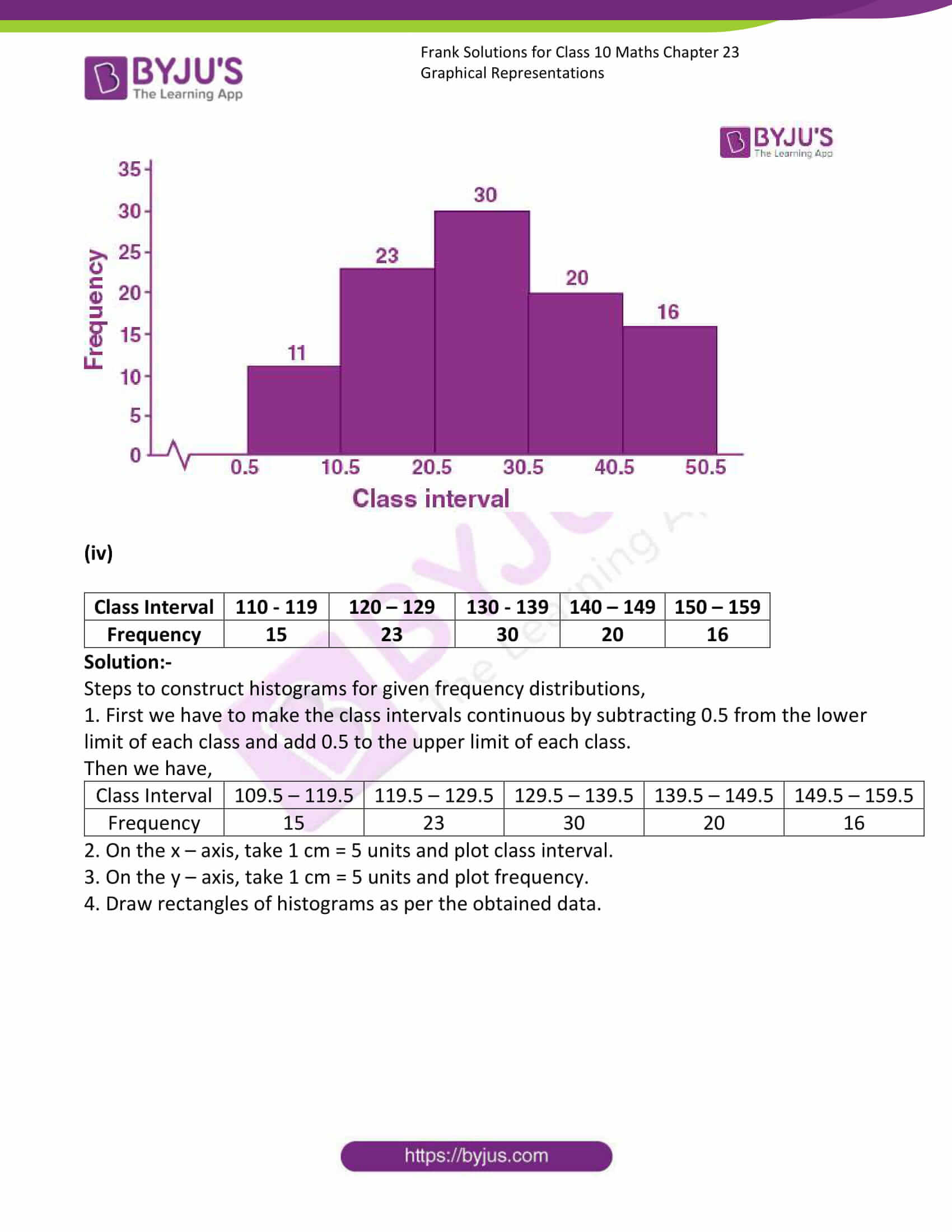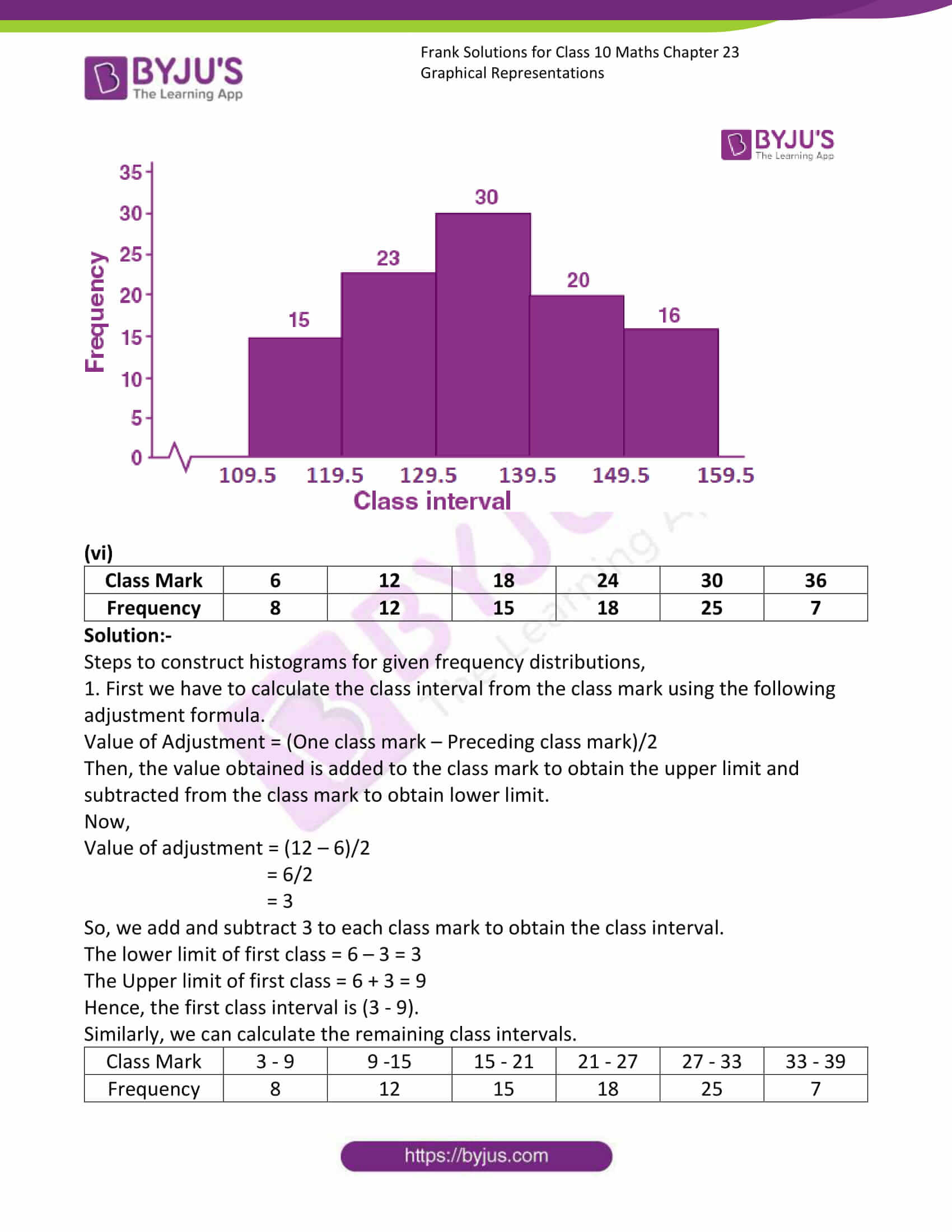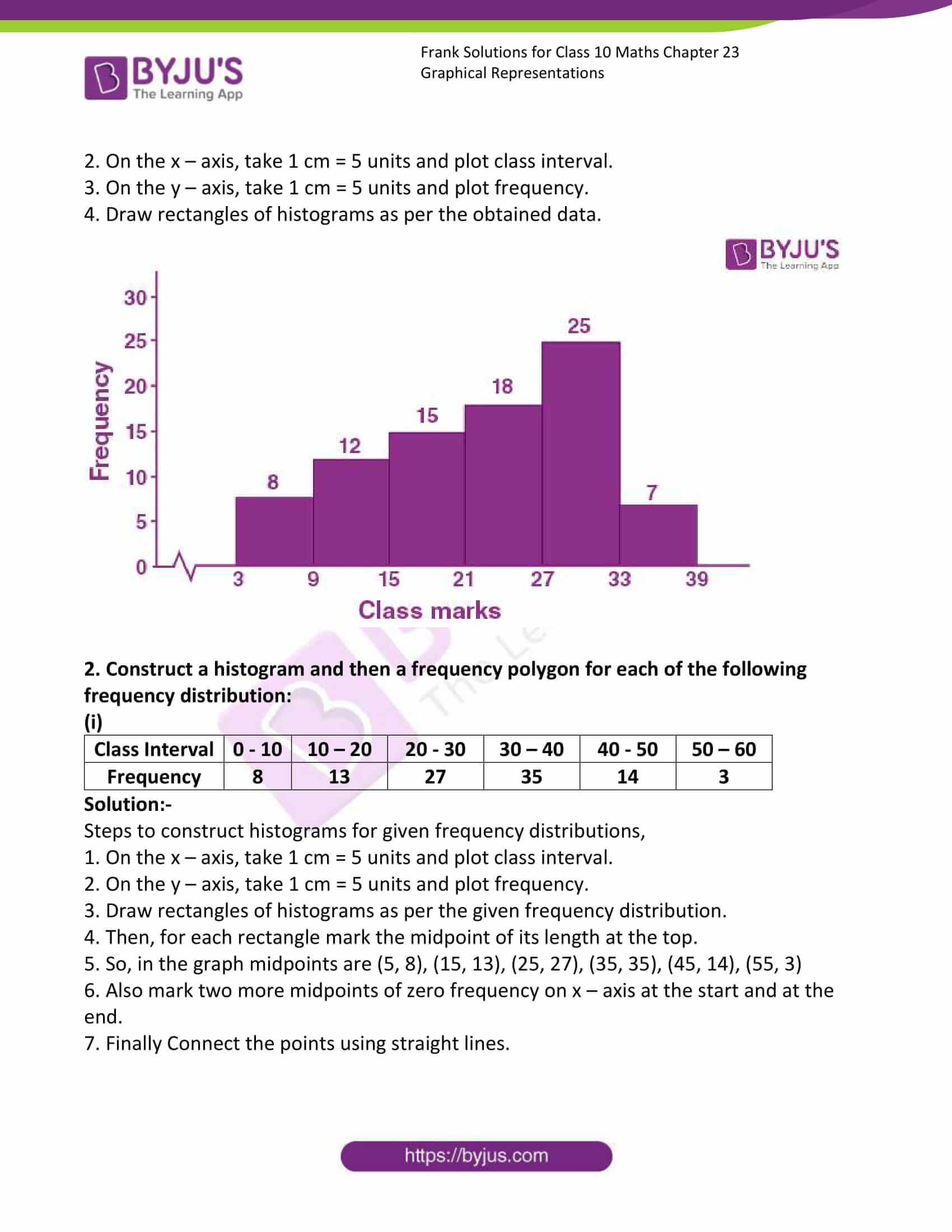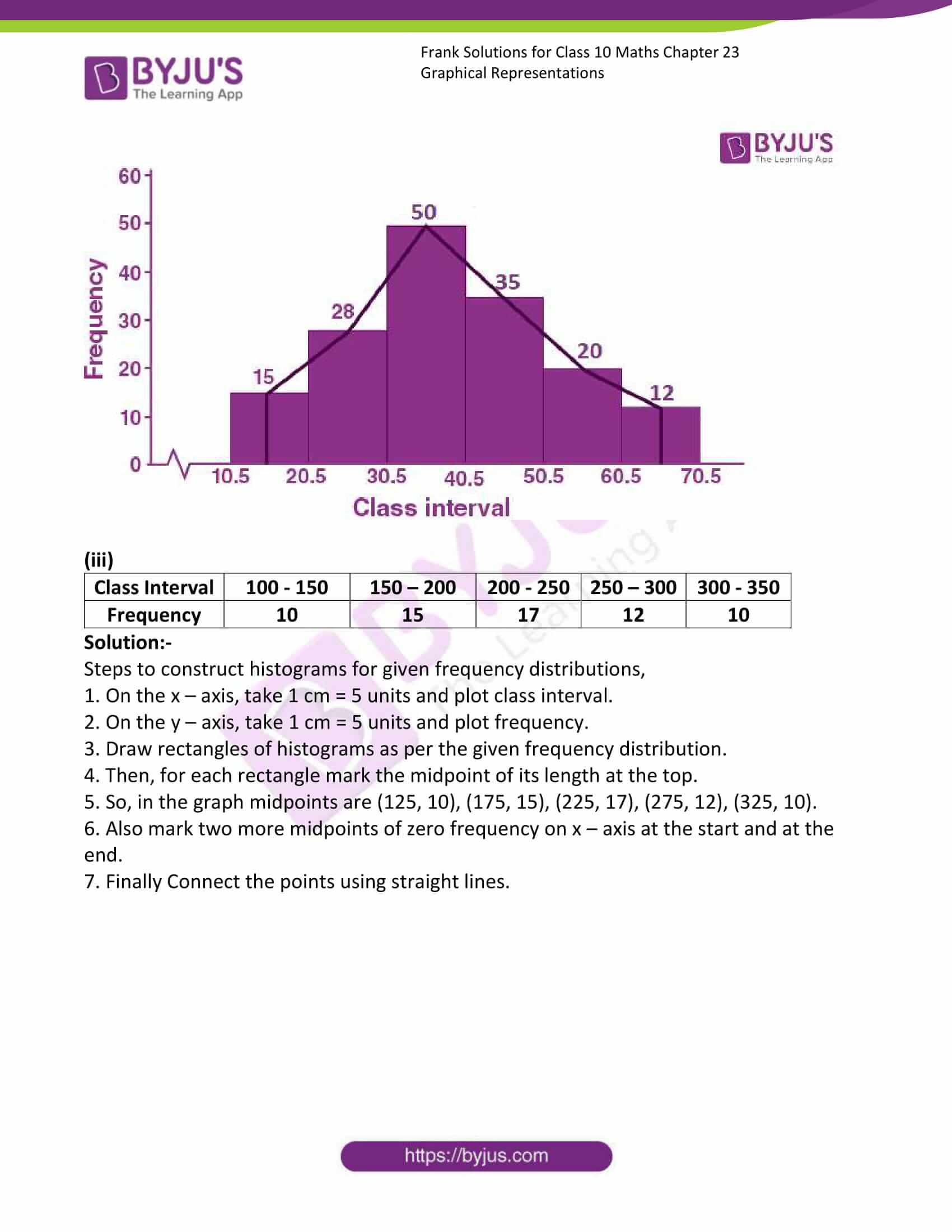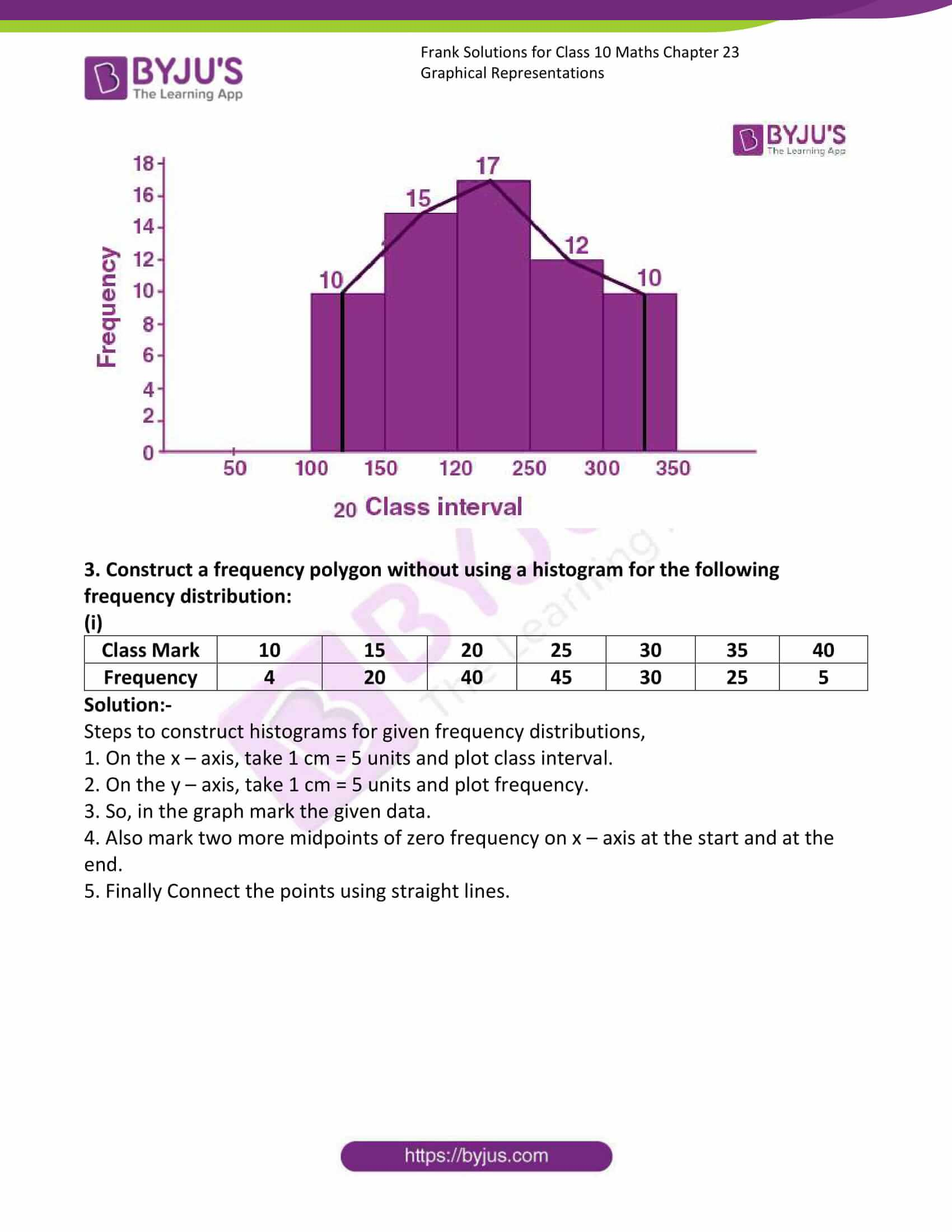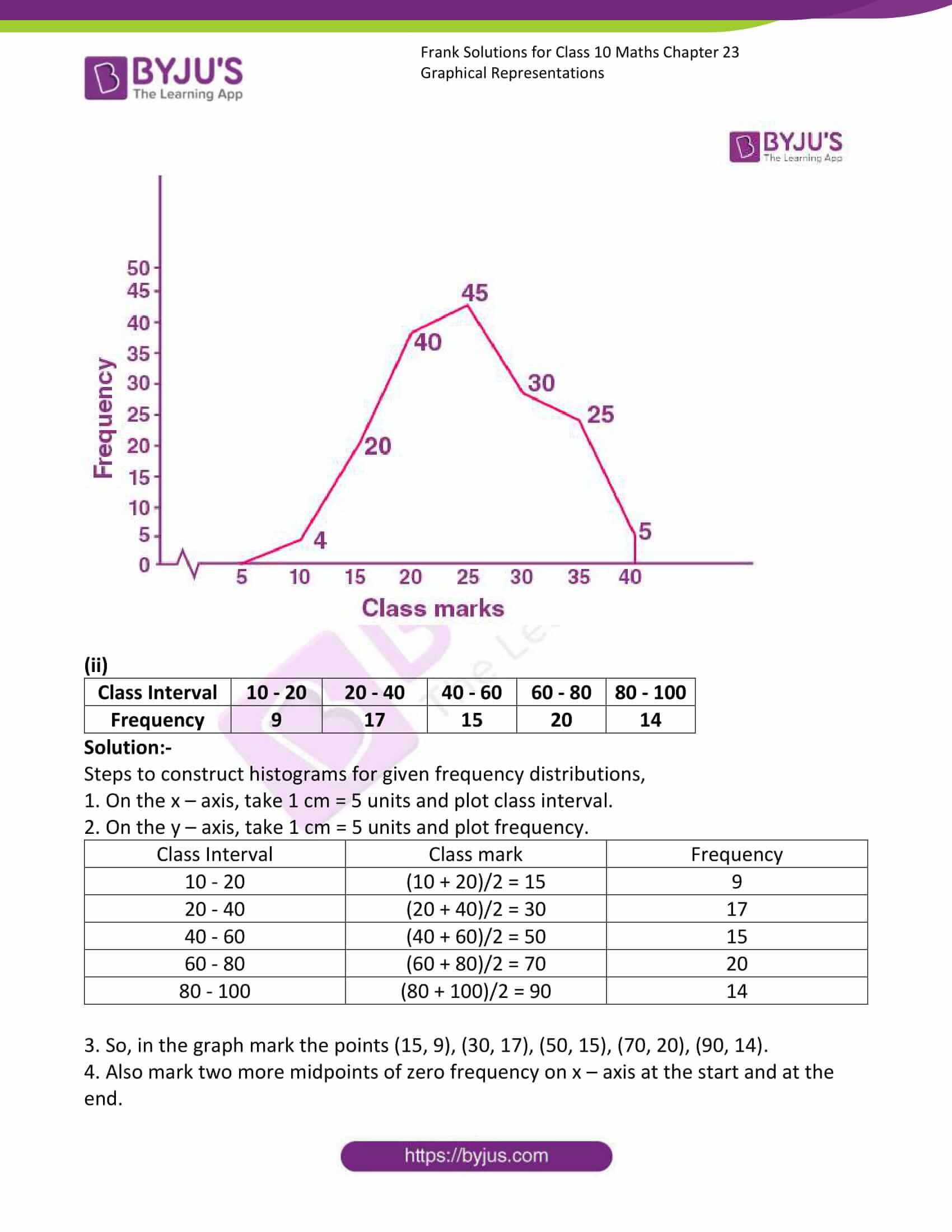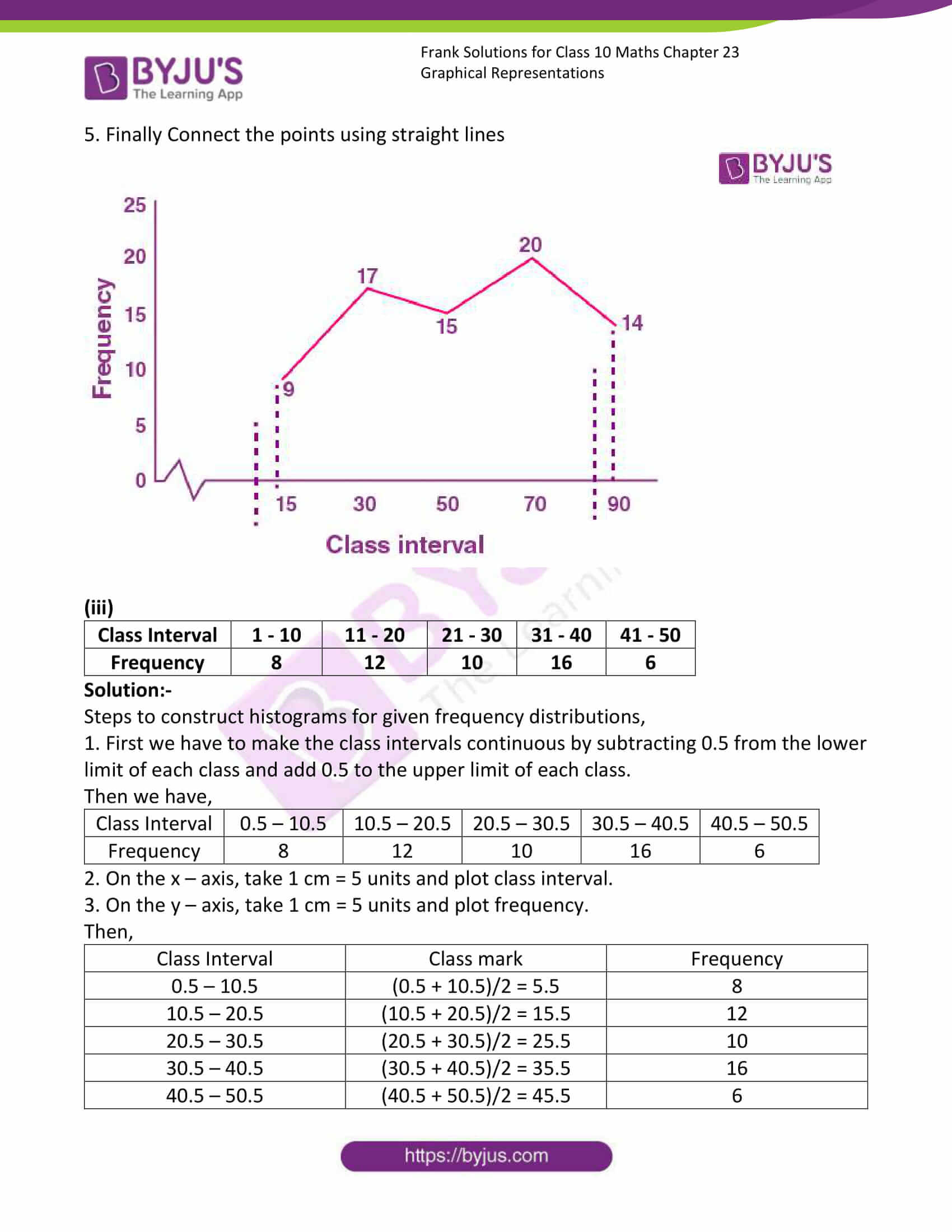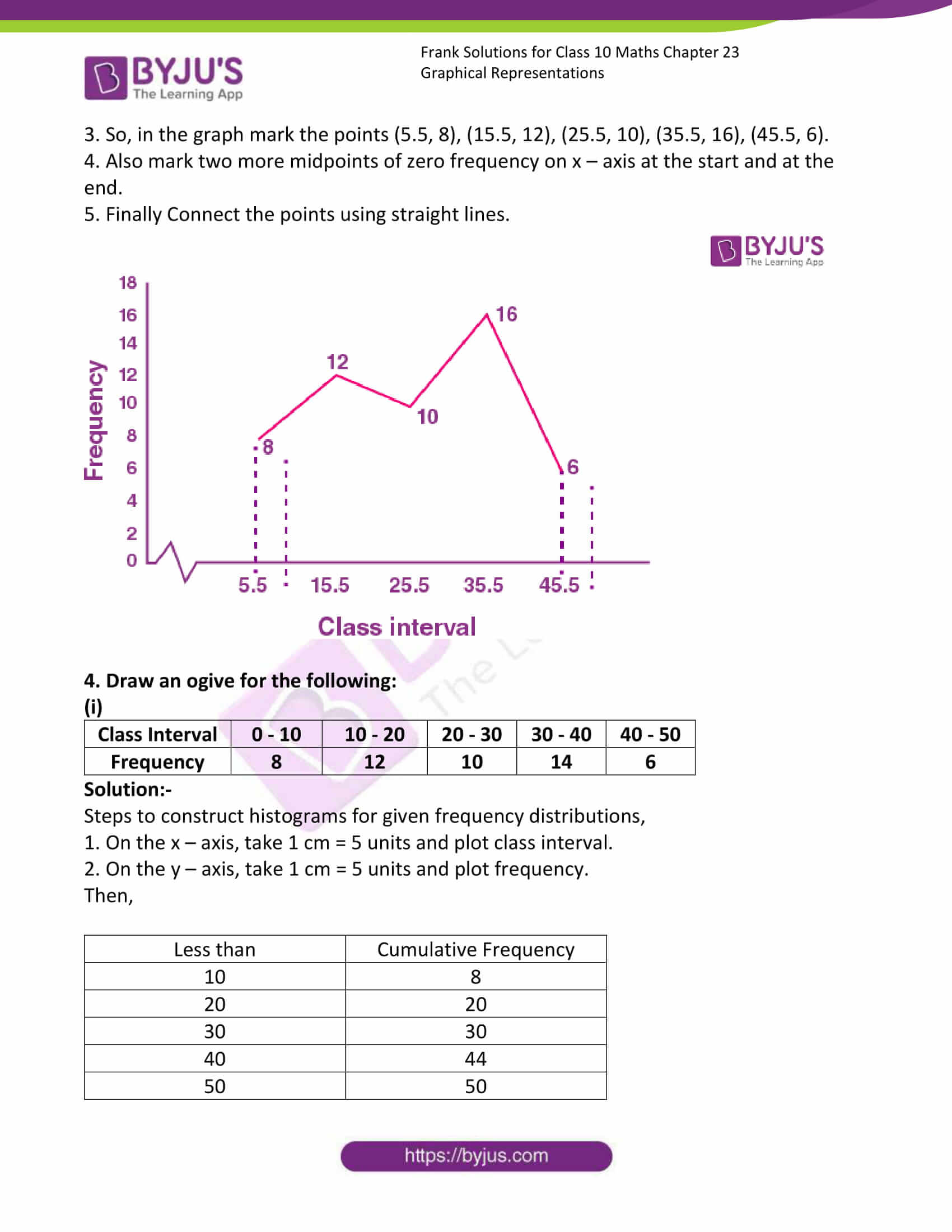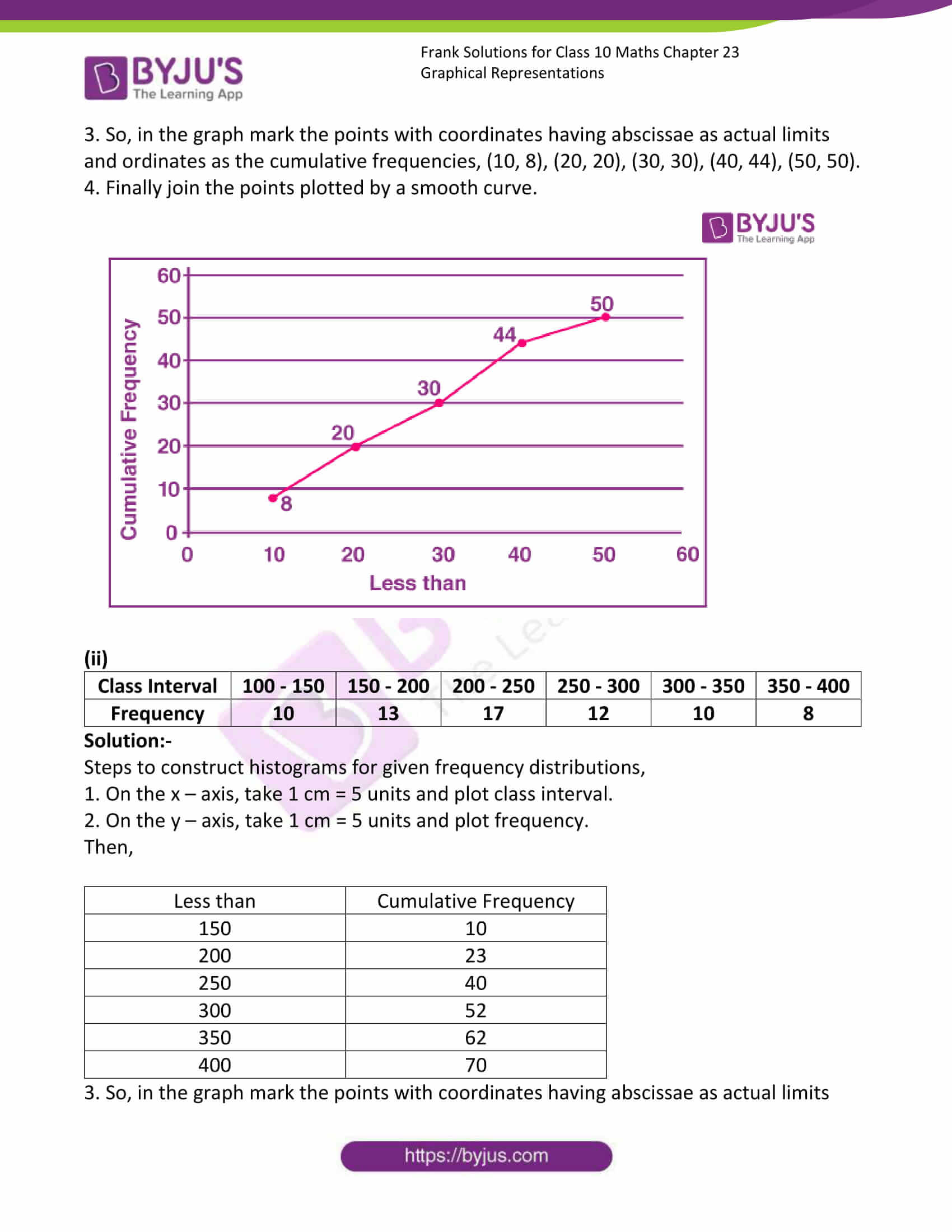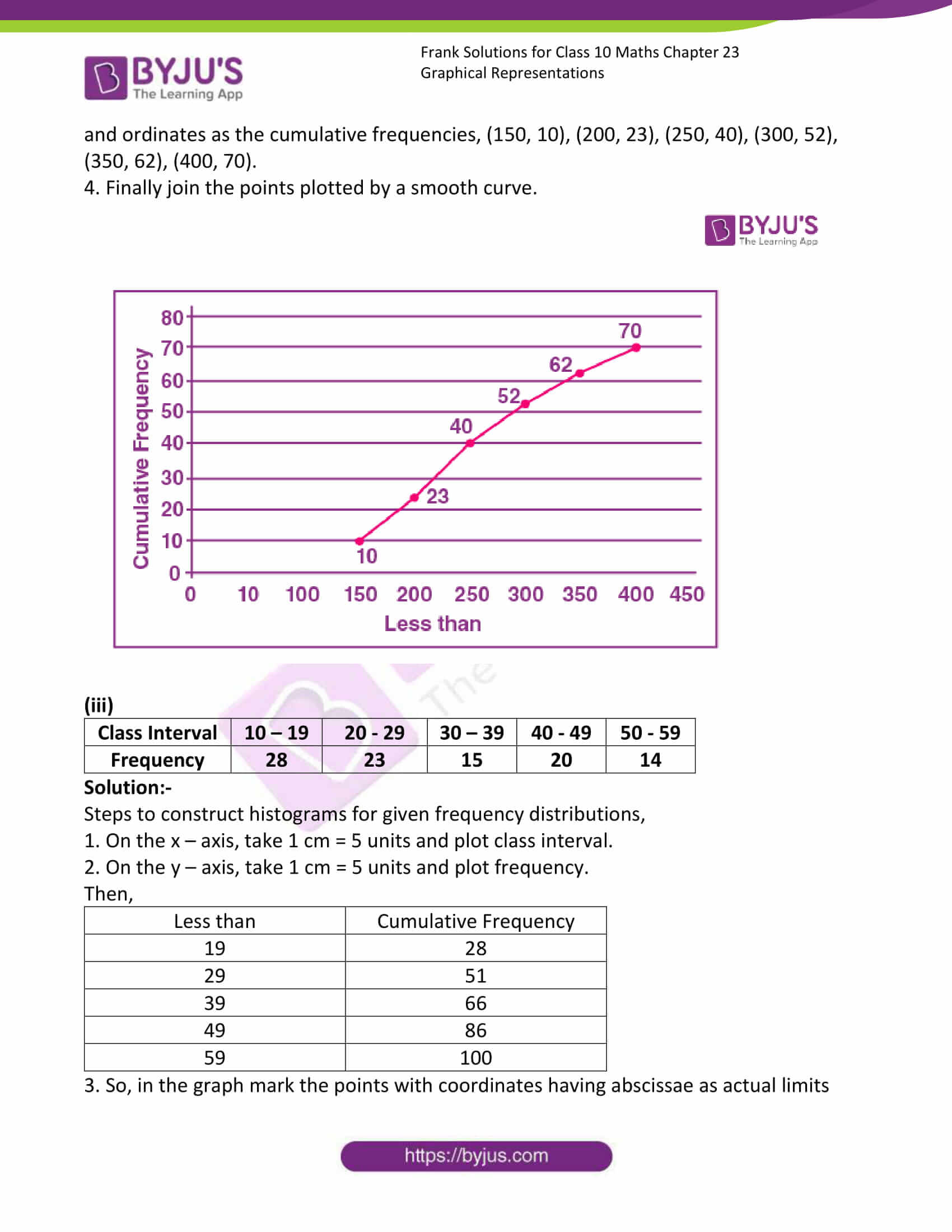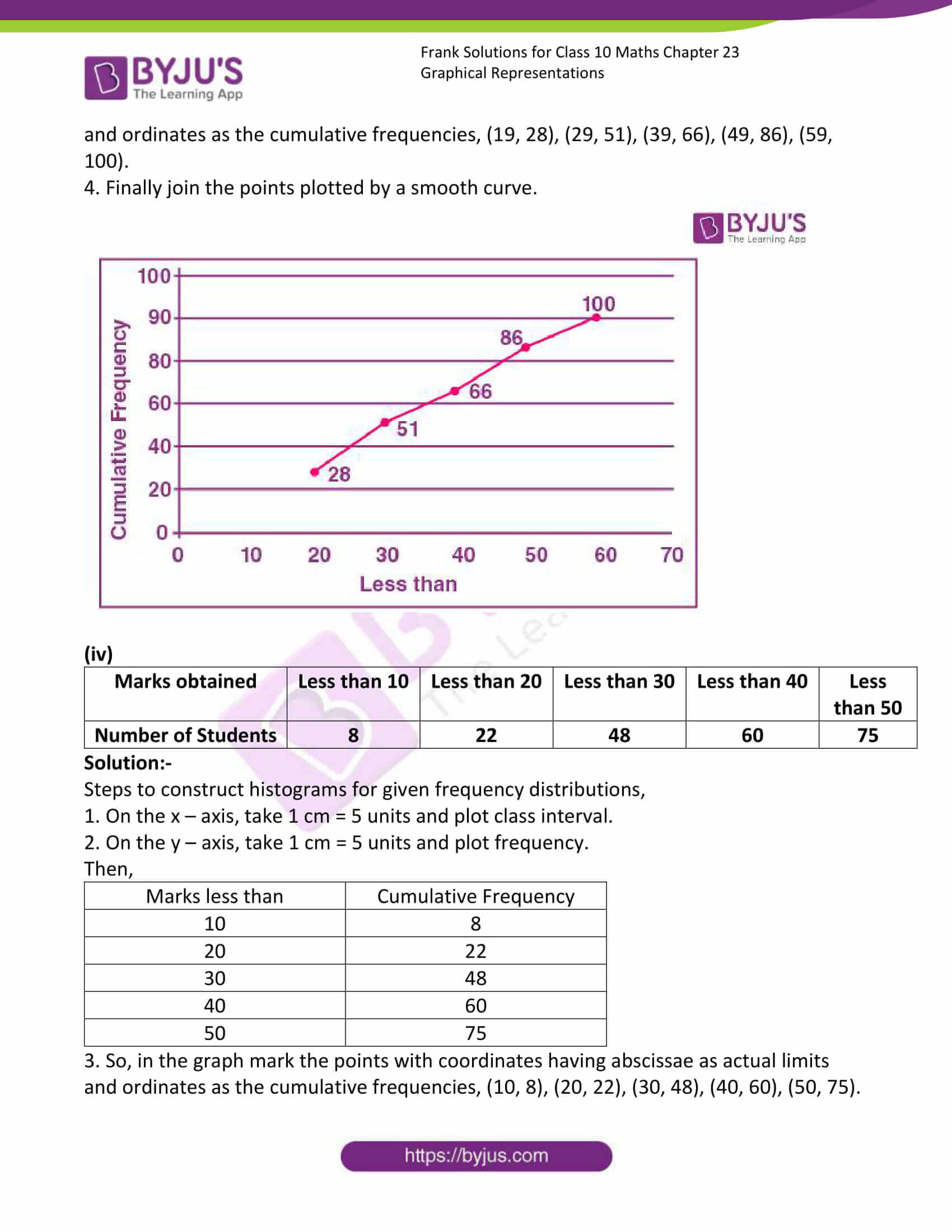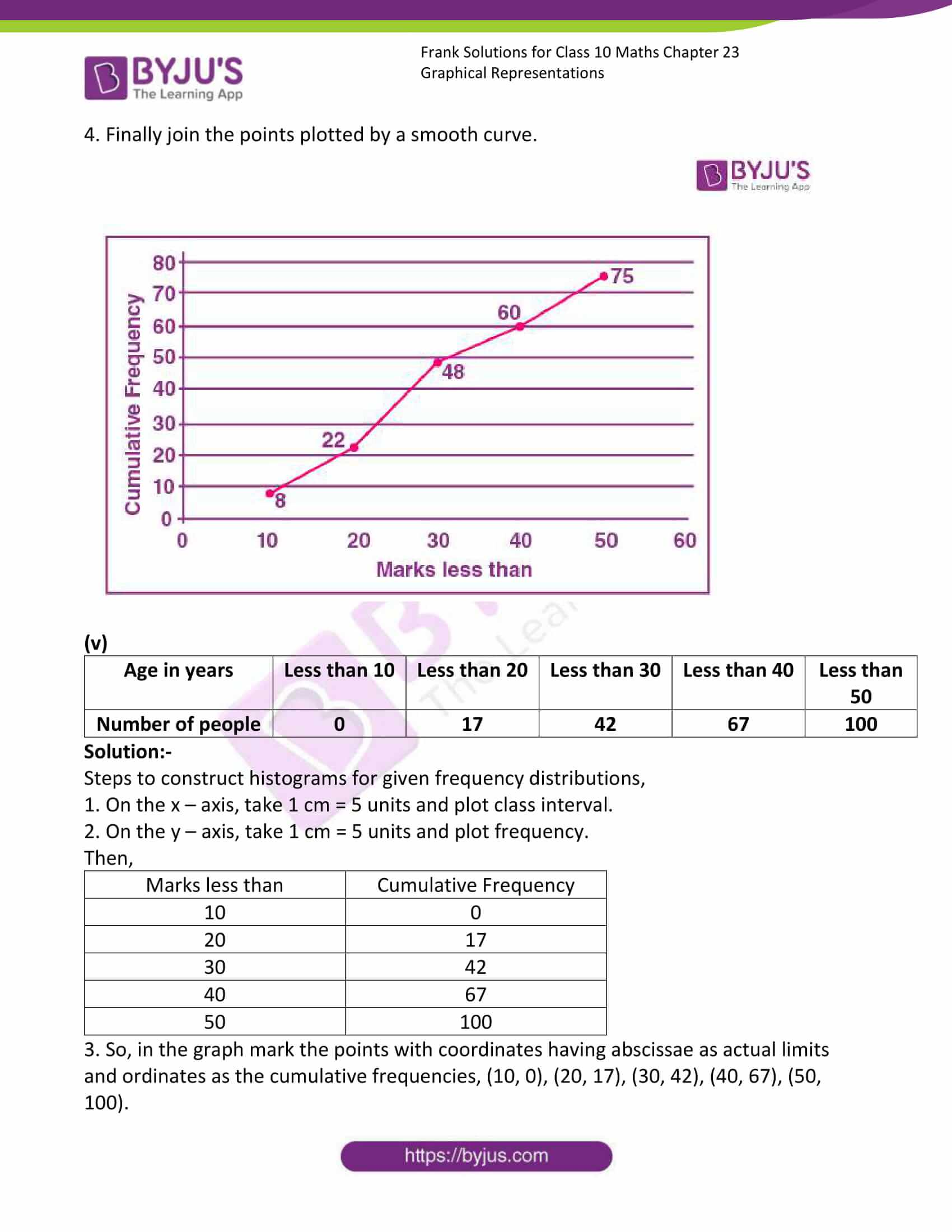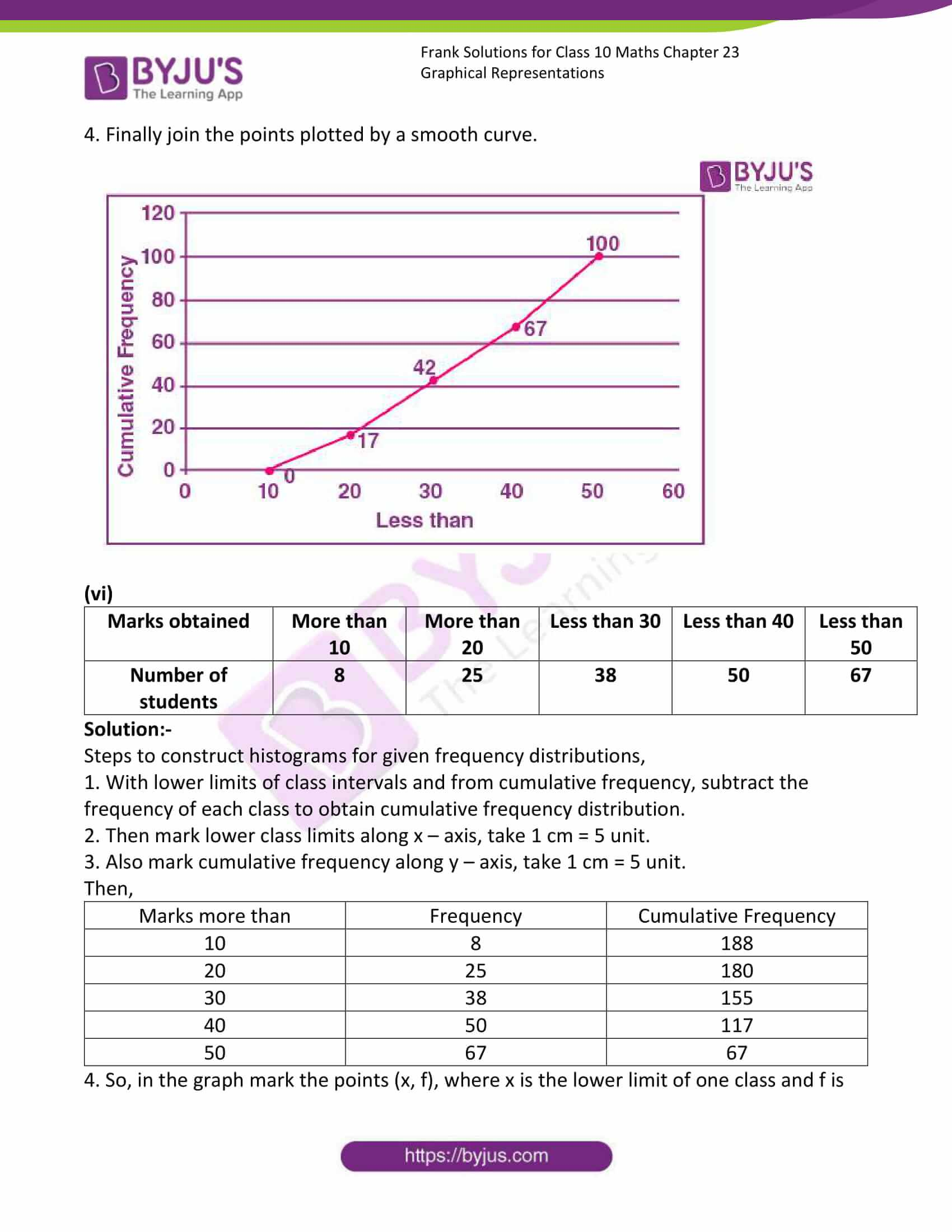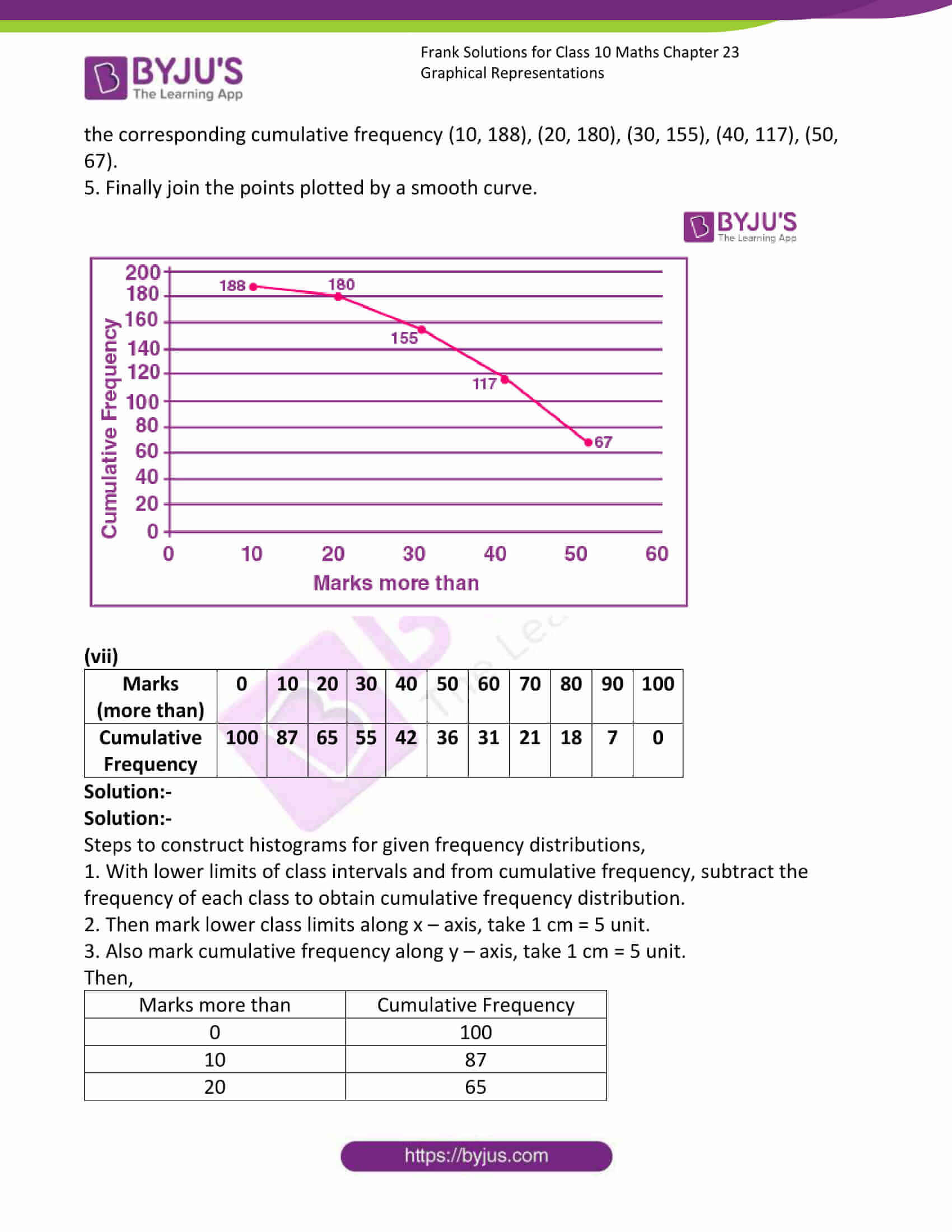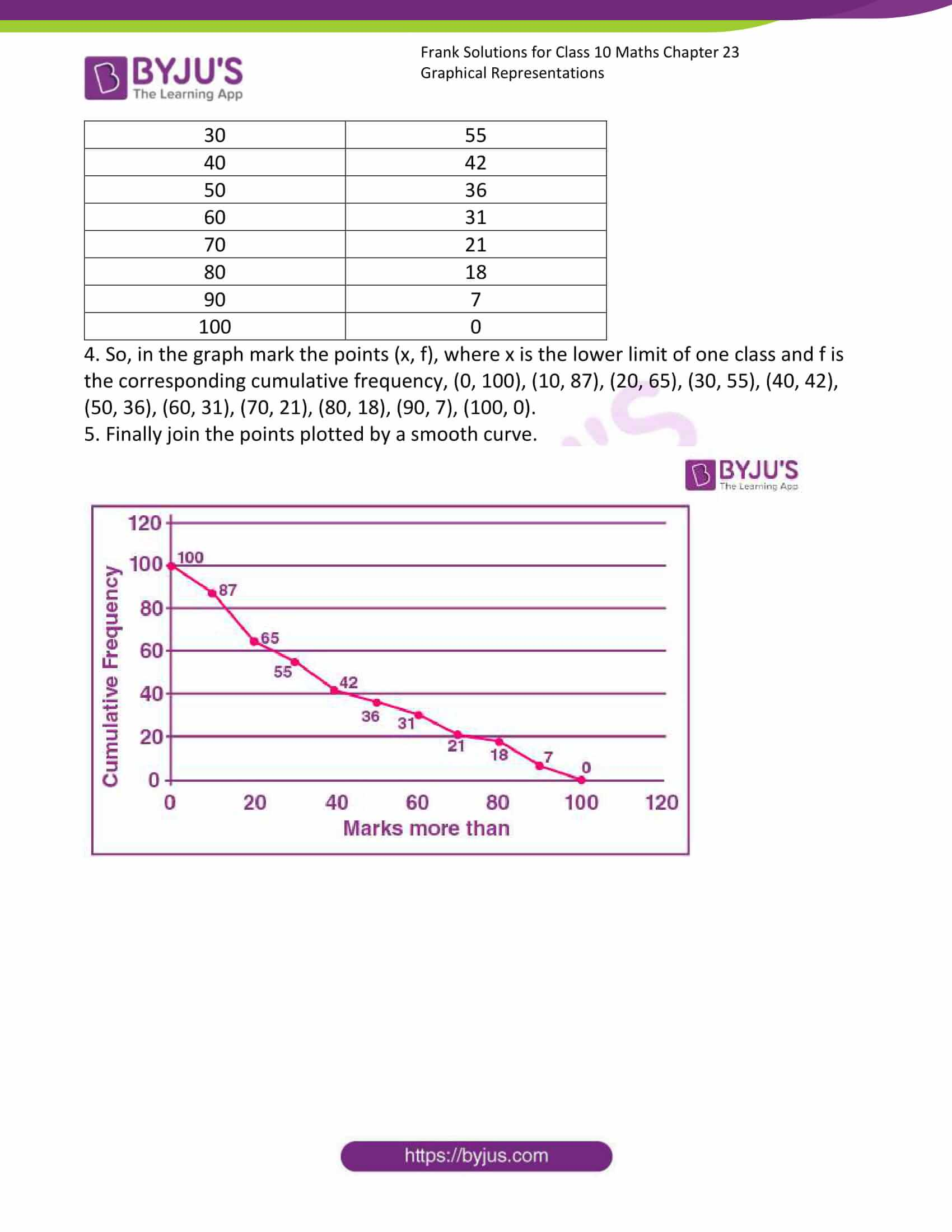### Access answers to Frank Solutions for Class 10 Maths Chapter 23 Graphical Representation

1. Construct histograms for each of the following frequency distributions:

(i)

 Class Interval 0 – 10 10 – 20 20 – 30 30 – 40 40 – 50 50 – 60 Frequency 8 20 34 22 10 6

Solution:-

Steps to construct histograms for given frequency distributions,

1. On the x – axis, take 1 cm = 5 units and plot class interval.

2. On the y – axis, take 1 cm = 5 units and plot frequency.

3. Draw rectangles of histograms as per the given frequency distribution.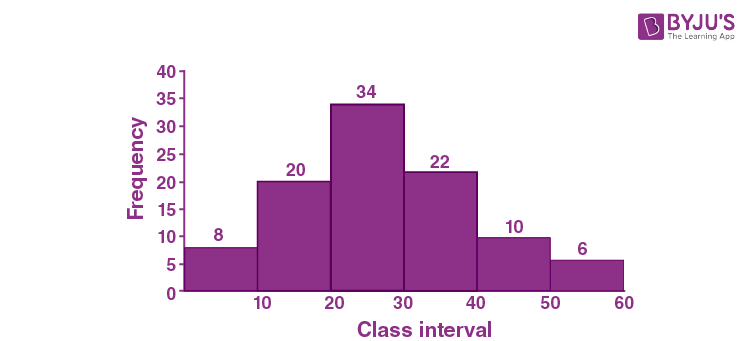(ii)

 Class Interval 130 – 140 140 – 150 150 – 160 160 – 170 170 – 180 Frequency 24 16 29 20 11

Solution:-

Steps to construct histograms for given frequency distributions,

1. On the x – axis, take 1 cm = 5 units and plot class interval.

2. On the y – axis, take 1 cm = 5 units and plot frequency.

3. Draw rectangles of histograms as per the given frequency distribution.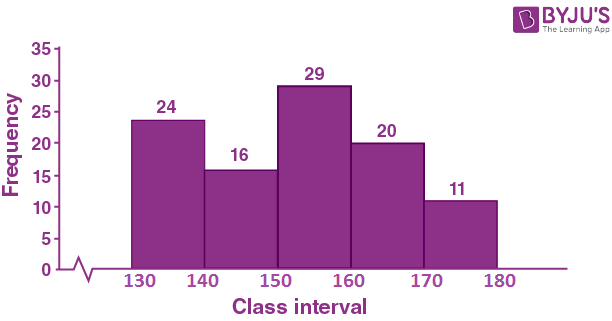(iii)

 Class Interval 1 – 10 11 – 20 21 – 30 31 – 40 41 – 50 Frequency 11 23 30 20 16

Solution:-

Steps to construct histograms for given frequency distributions,

1. First we have to make the class intervals continuous by subtracting 0.5 from the lower limit of each class and add 0.5 to the upper limit of each class.

Then we have,

 Class Interval 0.5 – 10.5 10.5 – 20.5 20.5 – 30.5 30.5 – 40.5 40.5 – 50.5 Frequency 11 23 30 20 16

2. On the x – axis, take 1 cm = 5 units and plot class interval.

3. On the y – axis, take 1 cm = 5 units and plot frequency.

4. Draw rectangles of histograms as per the obtained data.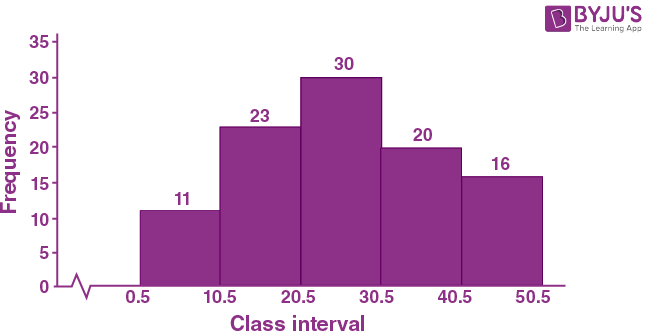(iv)

 Class Interval 110 – 119 120 – 129 130 – 139 140 – 149 150 – 159 Frequency 15 23 30 20 16

Solution:-

Steps to construct histograms for given frequency distributions,

1. First we have to make the class intervals continuous by subtracting 0.5 from the lower limit of each class and add 0.5 to the upper limit of each class.

Then we have,

 Class Interval 109.5 – 119.5 119.5 – 129.5 129.5 – 139.5 139.5 – 149.5 149.5 – 159.5 Frequency 15 23 30 20 16

2. On the x – axis, take 1 cm = 5 units and plot class interval.

3. On the y – axis, take 1 cm = 5 units and plot frequency.

4. Draw rectangles of histograms as per the obtained data.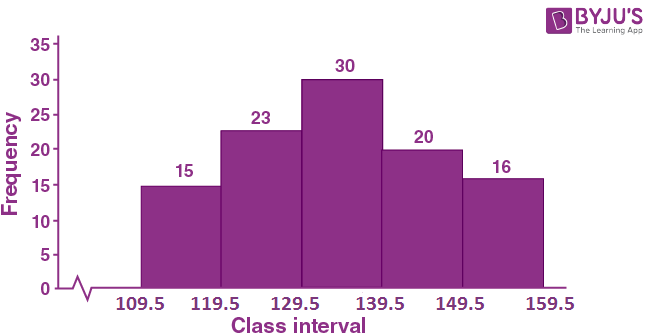(vi)

 Class Mark 6 12 18 24 30 36 Frequency 8 12 15 18 25 7

Solution:-

Steps to construct histograms for given frequency distributions,

1. First we have to calculate the class interval from the class mark using the following adjustment formula.

Value of Adjustment = (One class mark – Preceding class mark)/2

Then, the value obtained is added to the class mark to obtain the upper limit and subtracted from the class mark to obtain lower limit.

Now,

Value of adjustment = (12 – 6)/2

= 6/2

= 3

So, we add and subtract 3 to each class mark to obtain the class interval.

The lower limit of first class = 6 – 3 = 3

The Upper limit of first class = 6 + 3 = 9

Hence, the first class interval is (3 – 9).

Similarly, we can calculate the remaining class intervals.

 Class Mark 3 – 9 9 -15 15 – 21 21 – 27 27 – 33 33 – 39 Frequency 8 12 15 18 25 7

2. On the x – axis, take 1 cm = 5 units and plot class interval.

3. On the y – axis, take 1 cm = 5 units and plot frequency.

4. Draw rectangles of histograms as per the obtained data.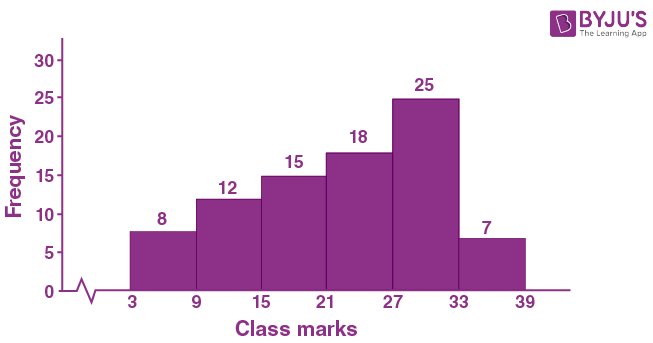2. Construct a histogram and then a frequency polygon for each of the following frequency distribution:

(i)

 Class Interval 0 – 10 10 – 20 20 – 30 30 – 40 40 – 50 50 – 60 Frequency 8 13 27 35 14 3

Solution:-

Steps to construct histograms for given frequency distributions,

1. On the x – axis, take 1 cm = 5 units and plot class interval.

2. On the y – axis, take 1 cm = 5 units and plot frequency.

3. Draw rectangles of histograms as per the given frequency distribution.

4. Then, for each rectangle mark the midpoint of its length at the top.

5. So, in the graph midpoints are (5, 8), (15, 13), (25, 27), (35, 35), (45, 14), (55, 3)

6. Also mark two more midpoints of zero frequency on x – axis at the start and at the end.

7. Finally Connect the points using straight lines.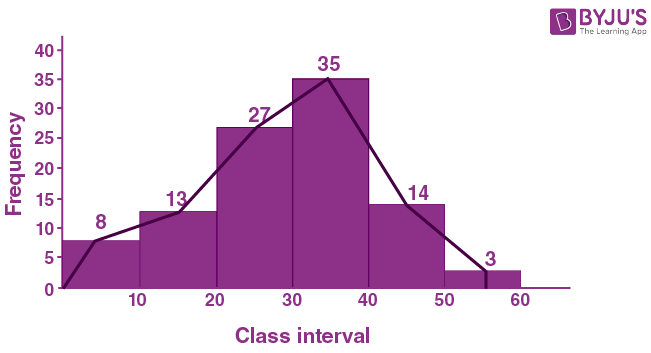(ii)

 Class Interval 11 – 20 21 – 30 31 – 40 41 – 50 51 – 60 61 – 70 Frequency 15 28 50 35 20 12

Solution:-

Steps to construct histograms for given frequency distributions,

1. First we have to make the class intervals continuous by subtracting 0.5 from the lower limit of each class and add 0.5 to the upper limit of each class.

Then we have,

 Class Interval 10.5 – 20.5 20.5 – 30.5 30.5 – 40.5 40.5 – 50.5 50.5 – 60.5 60.5 – 70.5 Frequency 15 28 50 35 20 12

2. On the x – axis, take 1 cm = 5 units and plot class interval.

3. On the y – axis, take 1 cm = 5 units and plot frequency.

4. Draw rectangles of histograms as per the given frequency distribution.

5. Then, for each rectangle mark the midpoint of its length at the top.

6. So, in the graph midpoints are (15.5, 15), (25.5, 28), (35.5, 50), (45.5, 35), (55.5, 20), (65.5, 12)

7. Also mark two more midpoints of zero frequency on x – axis at the start and at the end.

8. Finally Connect the points using straight lines.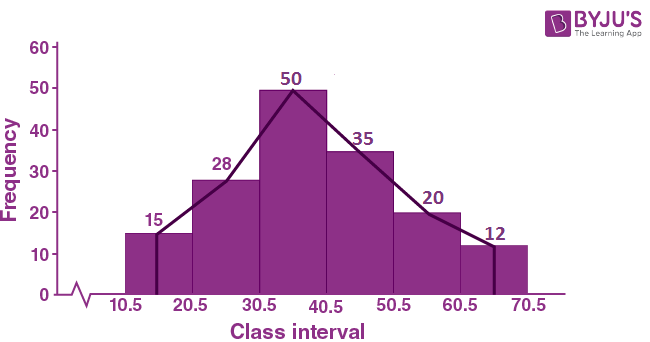(iii)

 Class Interval 100 – 150 150 – 200 200 – 250 250 – 300 300 – 350 Frequency 10 15 17 12 10

Solution:-

Steps to construct histograms for given frequency distributions,

1. On the x – axis, take 1 cm = 5 units and plot class interval.

2. On the y – axis, take 1 cm = 5 units and plot frequency.

3. Draw rectangles of histograms as per the given frequency distribution.

4. Then, for each rectangle mark the midpoint of its length at the top.

5. So, in the graph midpoints are (125, 10), (175, 15), (225, 17), (275, 12), (325, 10).

6. Also mark two more midpoints of zero frequency on x – axis at the start and at the end.

7. Finally Connect the points using straight lines.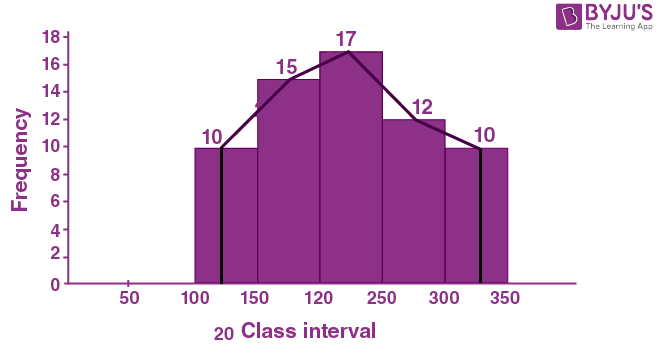3. Construct a frequency polygon without using a histogram for the following frequency distribution:

(i)

 Class Mark 10 15 20 25 30 35 40 Frequency 4 20 40 45 30 25 5

Solution:-

Steps to construct histograms for given frequency distributions,

1. On the x – axis, take 1 cm = 5 units and plot class interval.

2. On the y – axis, take 1 cm = 5 units and plot frequency.

3. So, in the graph mark the given data.

4. Also mark two more midpoints of zero frequency on x – axis at the start and at the end.

5. Finally Connect the points using straight lines.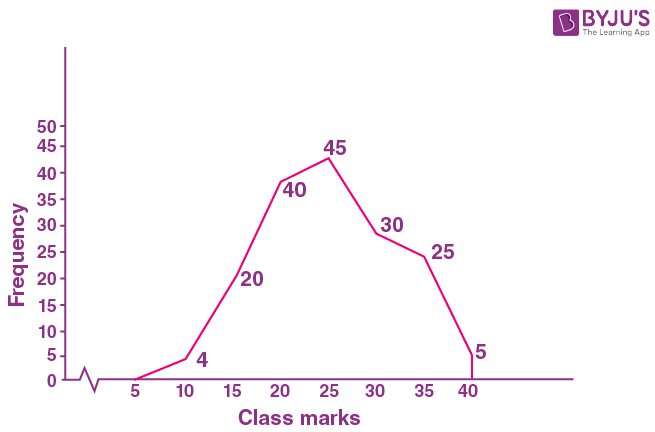(ii)

 Class Interval 10 – 20 20 – 40 40 – 60 60 – 80 80 – 100 Frequency 9 17 15 20 14

Solution:-

Steps to construct histograms for given frequency distributions,

1. On the x – axis, take 1 cm = 5 units and plot class interval.

2. On the y – axis, take 1 cm = 5 units and plot frequency.

 Class Interval Class mark Frequency 10 – 20 (10 + 20)/2 = 15 9 20 – 40 (20 + 40)/2 = 30 17 40 – 60 (40 + 60)/2 = 50 15 60 – 80 (60 + 80)/2 = 70 20 80 – 100 (80 + 100)/2 = 90 14

3. So, in the graph mark the points (15, 9), (30, 17), (50, 15), (70, 20), (90, 14).

4. Also mark two more midpoints of zero frequency on x – axis at the start and at the end.

5. Finally Connect the points using straight lines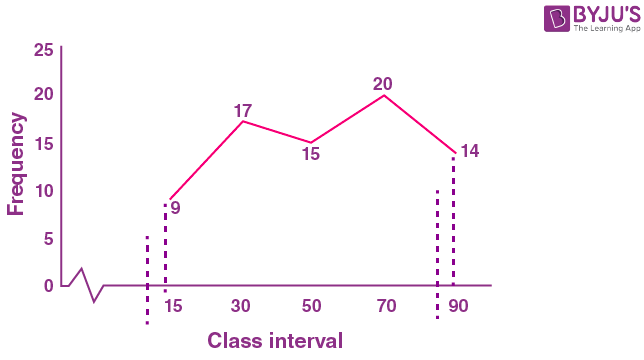(iii)

 Class Interval 1 – 10 11 – 20 21 – 30 31 – 40 41 – 50 Frequency 8 12 10 16 6

Solution:-

Steps to construct histograms for given frequency distributions,

1. First we have to make the class intervals continuous by subtracting 0.5 from the lower limit of each class and add 0.5 to the upper limit of each class.

Then we have,

 Class Interval 0.5 – 10.5 10.5 – 20.5 20.5 – 30.5 30.5 – 40.5 40.5 – 50.5 Frequency 8 12 10 16 6

2. On the x – axis, take 1 cm = 5 units and plot class interval.

3. On the y – axis, take 1 cm = 5 units and plot frequency.

Then,

 Class Interval Class mark Frequency 0.5 – 10.5 (0.5 + 10.5)/2 = 5.5 8 10.5 – 20.5 (10.5 + 20.5)/2 = 15.5 12 20.5 – 30.5 (20.5 + 30.5)/2 = 25.5 10 30.5 – 40.5 (30.5 + 40.5)/2 = 35.5 16 40.5 – 50.5 (40.5 + 50.5)/2 = 45.5 6

3. So, in the graph mark the points (5.5, 8), (15.5, 12), (25.5, 10), (35.5, 16), (45.5, 6).

4. Also mark two more midpoints of zero frequency on x – axis at the start and at the end.

5. Finally Connect the points using straight lines.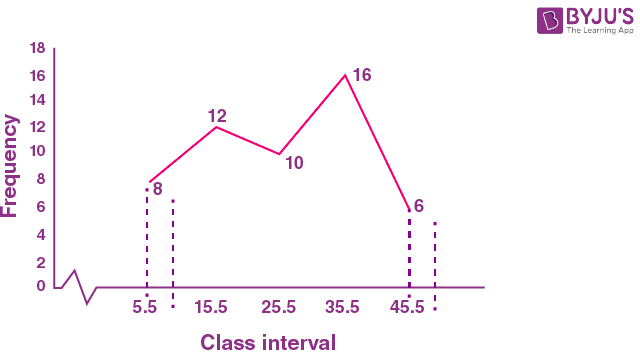4. Draw an ogive for the following:

(i)

 Class Interval 0 – 10 10 – 20 20 – 30 30 – 40 40 – 50 Frequency 8 12 10 14 6

Solution:-

Steps to construct histograms for given frequency distributions,

1. On the x – axis, take 1 cm = 5 units and plot class interval.

2. On the y – axis, take 1 cm = 5 units and plot frequency.

Then,

 Less than Cumulative Frequency 10 8 20 20 30 30 40 44 50 50

3. So, in the graph mark the points with coordinates having abscissae as actual limits and ordinates as the cumulative frequencies, (10, 8), (20, 20), (30, 30), (40, 44), (50, 50).

4. Finally join the points plotted by a smooth curve.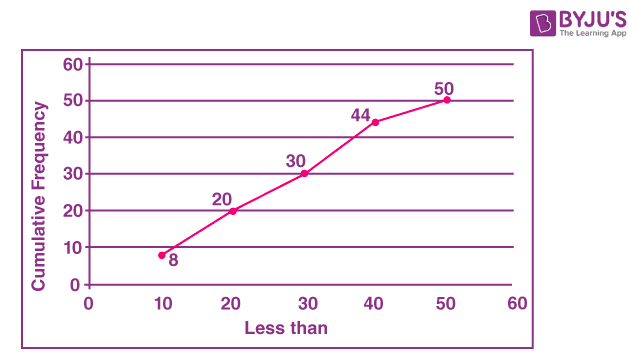(ii)

 Class Interval 100 – 150 150 – 200 200 – 250 250 – 300 300 – 350 350 – 400 Frequency 10 13 17 12 10 8

Solution:-

Steps to construct histograms for given frequency distributions,

1. On the x – axis, take 1 cm = 5 units and plot class interval.

2. On the y – axis, take 1 cm = 5 units and plot frequency.

Then,

 Less than Cumulative Frequency 150 10 200 23 250 40 300 52 350 62 400 70

3. So, in the graph mark the points with coordinates having abscissae as actual limits and ordinates as the cumulative frequencies, (150, 10), (200, 23), (250, 40), (300, 52), (350, 62), (400, 70).

4. Finally join the points plotted by a smooth curve.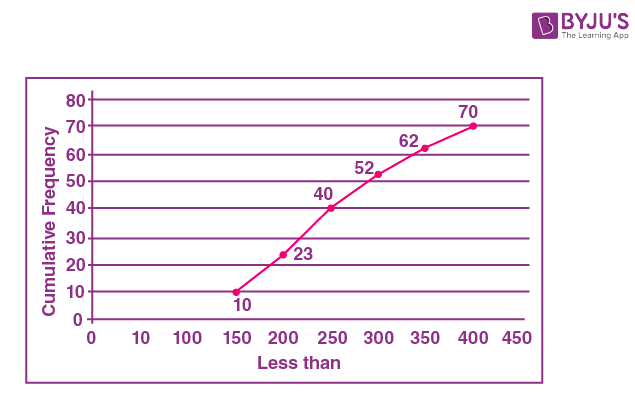(iii)

 Class Interval 10 – 19 20 – 29 30 – 39 40 – 49 50 – 59 Frequency 28 23 15 20 14

Solution:-

Steps to construct histograms for given frequency distributions,

1. On the x – axis, take 1 cm = 5 units and plot class interval.

2. On the y – axis, take 1 cm = 5 units and plot frequency.

Then,

 Less than Cumulative Frequency 19 28 29 51 39 66 49 86 59 100

3. So, in the graph mark the points with coordinates having abscissae as actual limits and ordinates as the cumulative frequencies, (19, 28), (29, 51), (39, 66), (49, 86), (59, 100).

4. Finally join the points plotted by a smooth curve.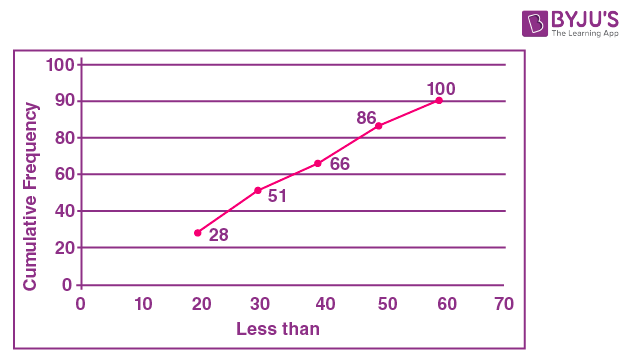(iv)

 Marks obtained Less than 10 Less than 20 Less than 30 Less than 40 Less than 50 Number of Students 8 22 48 60 75

Solution:-

Steps to construct histograms for given frequency distributions,

1. On the x – axis, take 1 cm = 5 units and plot class interval.

2. On the y – axis, take 1 cm = 5 units and plot frequency.

Then,

 Marks less than Cumulative Frequency 10 8 20 22 30 48 40 60 50 75

3. So, in the graph mark the points with coordinates having abscissae as actual limits and ordinates as the cumulative frequencies, (10, 8), (20, 22), (30, 48), (40, 60), (50, 75).

4. Finally join the points plotted by a smooth curve.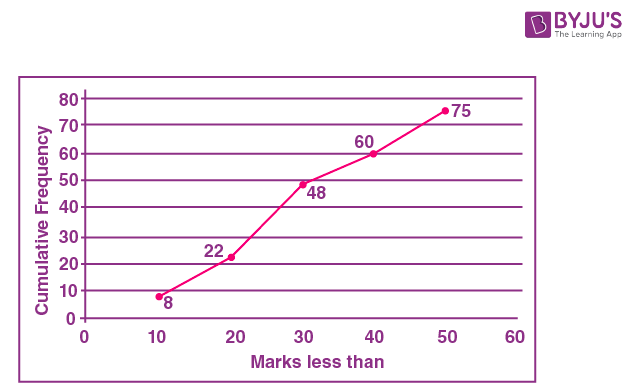(v)

 Age in years Less than 10 Less than 20 Less than 30 Less than 40 Less than 50 Number of people 0 17 42 67 100

Solution:-

Steps to construct histograms for given frequency distributions,

1. On the x – axis, take 1 cm = 5 units and plot class interval.

2. On the y – axis, take 1 cm = 5 units and plot frequency.

Then,

 Marks less than Cumulative Frequency 10 0 20 17 30 42 40 67 50 100

3. So, in the graph mark the points with coordinates having abscissae as actual limits and ordinates as the cumulative frequencies, (10, 0), (20, 17), (30, 42), (40, 67), (50, 100).

4. Finally join the points plotted by a smooth curve.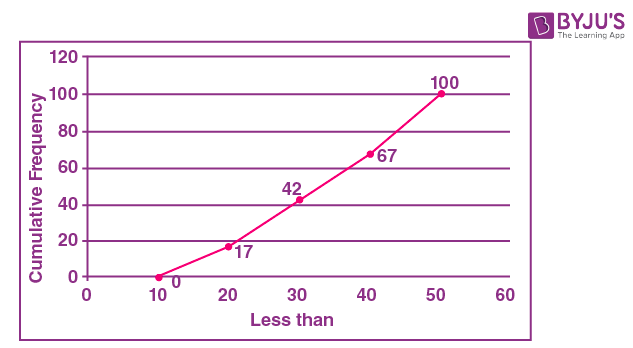(vi)

 Marks obtained More than 10 More than 20 Less than 30 Less than 40 Less than 50 Number of students 8 25 38 50 67

Solution:-

Steps to construct histograms for given frequency distributions,

1. With lower limits of class intervals and from cumulative frequency, subtract the frequency of each class to obtain cumulative frequency distribution.

2. Then mark lower class limits along x – axis, take 1 cm = 5 unit.

3. Also mark cumulative frequency along y – axis, take 1 cm = 5 unit.

Then,

 Marks more than Frequency Cumulative Frequency 10 8 188 20 25 180 30 38 155 40 50 117 50 67 67

4. So, in the graph mark the points (x, f), where x is the lower limit of one class and f is the corresponding cumulative frequency (10, 188), (20, 180), (30, 155), (40, 117), (50, 67).

5. Finally join the points plotted by a smooth curve.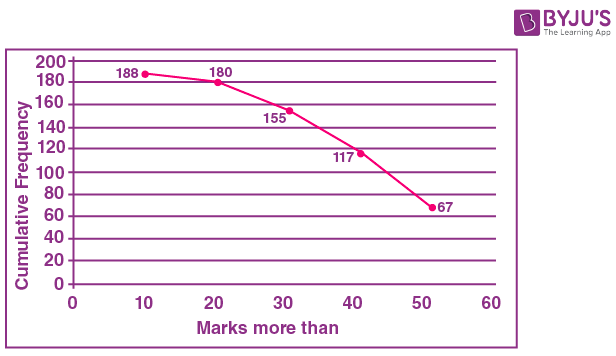(vii)

 Marks (more than) 0 10 20 30 40 50 60 70 80 90 100 Cumulative Frequency 100 87 65 55 42 36 31 21 18 7 0

Solution:-

Solution:-

Steps to construct histograms for given frequency distributions,

1. With lower limits of class intervals and from cumulative frequency, subtract the frequency of each class to obtain cumulative frequency distribution.

2. Then mark lower class limits along x – axis, take 1 cm = 5 unit.

3. Also mark cumulative frequency along y – axis, take 1 cm = 5 unit.

Then,

 Marks more than Cumulative Frequency 0 100 10 87 20 65 30 55 40 42 50 36 60 31 70 21 80 18 90 7 100 0

4. So, in the graph mark the points (x, f), where x is the lower limit of one class and f is the corresponding cumulative frequency, (0, 100), (10, 87), (20, 65), (30, 55), (40, 42), (50, 36), (60, 31), (70, 21), (80, 18), (90, 7), (100, 0).

5. Finally join the points plotted by a smooth curve.